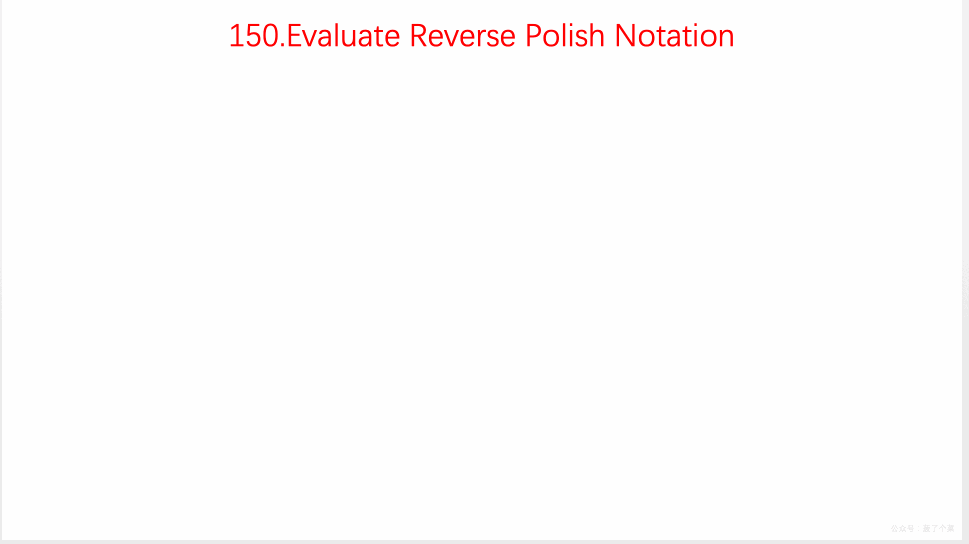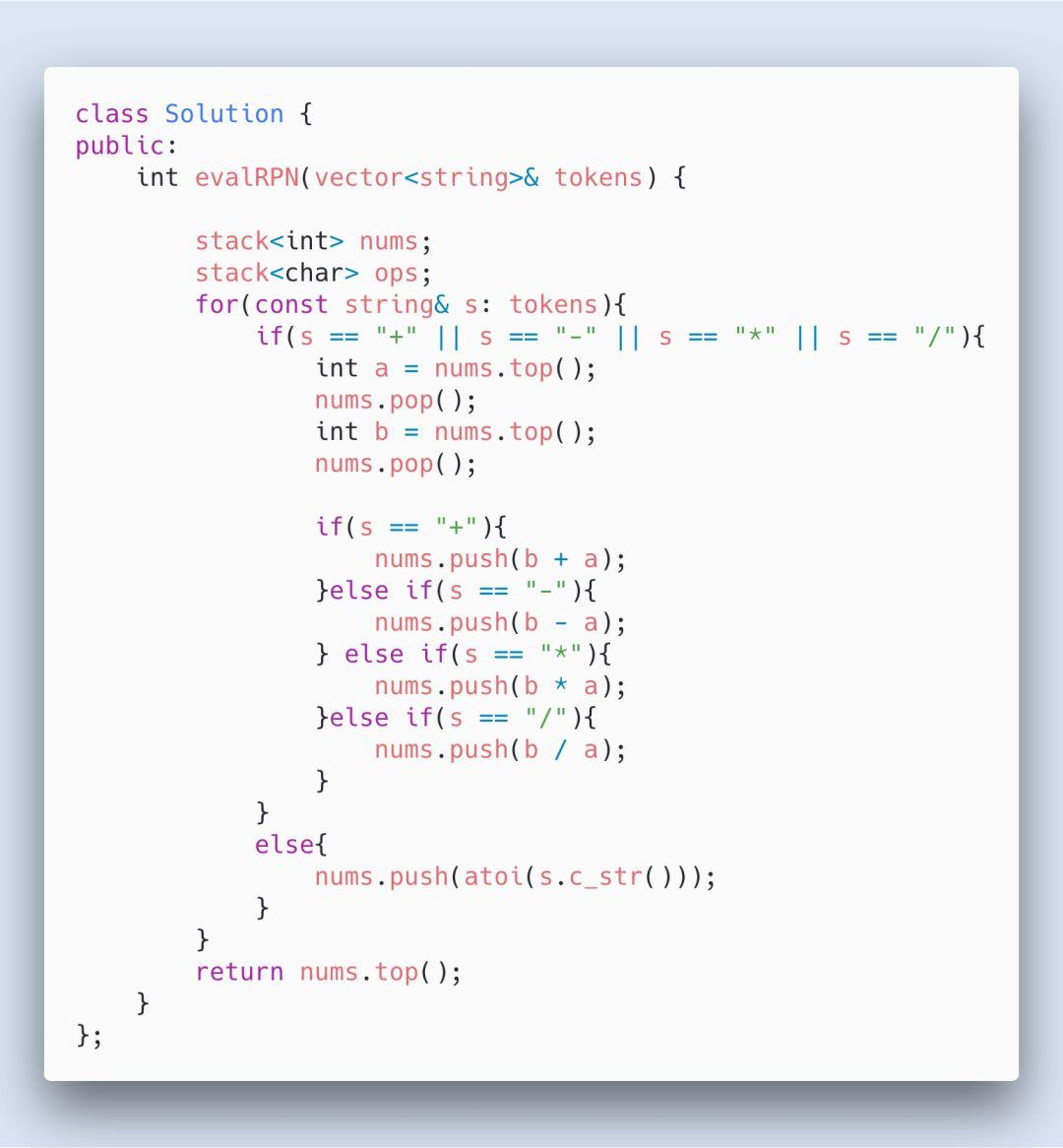LeetCode上第150号问题：逆波兰表达式求值

## 题目

输入: [“2”, “1”, “+”, “3”, “*”]
输出: 9
解释: ((2 + 1) * 3) = 9

示例 2：

输入: [“10”, “6”, “9”, “3”, “+”, “-11”, “*”, “/”, “*”, “17”, “+”, “5”, “+”]
输出: 22
解释:

((10 * (6 / ((9 + 3) * -11))) + 17) + 5

= ((10 * (6 / (12 * -11))) + 17) + 5

= ((10 * (6 / -132)) + 17) + 5

= ((10 * 0) + 17) + 5

= (0 + 17) + 5

= 17 + 5

= 22

## 解题思路

• 从前往后遍历数组

• 遇到数字则压入栈中

• 遇到符号，则把栈顶的两个数字拿出来运算，把结果再压入栈中

• 遍历完整个数组，栈顶数字即为最终答案

## 动画演示## 参考代码